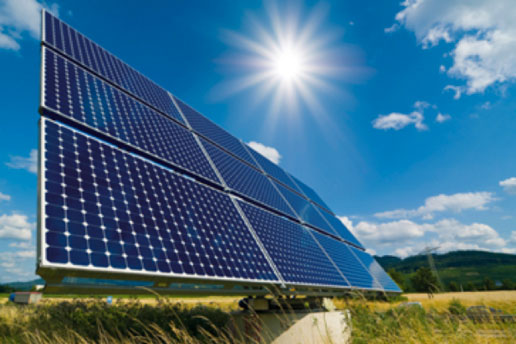# Experimental Verification of Joule's Law of HeatingJoule in 1841 experimentally studied the heating effect of electric current. He found that the amount of heat, H produce in a conductor due to the flow of current,

(i)  is directly proportional to the square of the current flowing through the conductor i.e. HI

(ii) is directly proportional to the resistance across the conductor, HR

(iii)is directly proportional to time for which the current is passed, Ht

Mathematically,

HIRT

H= IRT/J

Where J is called Joule’s mechanical equivalent of heat. Its value is 1/4.2 calorie(cal)

And it is a conversion factor only

Derivation

Expression of heat developed in a wire

When an electric charge Q moves against a p.d. V, the amount of work done is given by

W=Q×V                           ------------(1)

We know, current, I=Q/t

Q=I×t                               ------------(2)

By ohm's law,

V=I×R                             ------------(3)

Putting eqs. (2) and (3) in eq. (1),

W=Q×V

W=I×t×I×R
W=I²
Rt

Assuming that all the electrical work done is converted into heat energy,(taking wire as passive resistor ), we get
Heat produced , H=I
2Rt joules
This relation is known as Joule's law of heating.